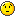# Superimpose 6 existing histograms

Hi!

I have already made six histograms and they are stored in six different .root files, located not in root directory. I wanted to know how can I superimpose all of them and add a legend to each of them (by writing a macro.C file or just using root terminal).

Can anyone help?

Load each of them into memory (by opening the different TFiles and Get each one). Create a THStack to put them in, then draw the THStack with the “nostack” option. The gPad should have a useful BuildLegend method, or make a TLegend object and add each of the histograms to it manually. Then draw the TLegend.

Jean-François

How do you get each one?

I write the following:

[code]- TFile f(“dir_of_the_root_file_”);

• TH1F h1 = (TH1F)f.Get(“p1”);[/code]

But this last step gives the following error:

``` Error: Symbol f is not defined in current scope (tmpfile):1:```

Can anyone help?

Also, I am using this THStack (within canvas) code:

[code]TCanvas *hstack() {

THStack *hs = new THStack(“hs”,“Stacked 1D histograms”);
h1>SetFillColor(kRed);
h1->SetMarkerColor(kRed);

TCanvas *cst = new TCanvas(“cst”,“stacked hists”,0, 0, 1000, 1000);

//the parameters below are optional and I will edit them when I can run the THStack this code.
cst->SetFillColor(41);
cst->Divide(2,2);
cst->cd(1);
hs->Draw();
cst->cd(2);
hs->Draw(“nostack,e1p”);
cst->cd(3);
hs->Draw(“lego1”);
{[/code]

If anything seems suspicious on the code I wrote let me know.
Thank you.

It is hard to tell what goes wrong in your TFile command as you do not show the real command you performed. You should do something like:

``````TFile f("file1.root");
TH1F *h1 = (TH1F*)f.Get("h1");

TFile f("file2.root");
TH1F *h2 = (TH1F*)f.Get("h2");

TFile f("file3.root");
TH1F *h3 = (TH1F*)f.Get("h3");

THStack *hs = new THStack("hs","Stacked 1D histograms");

hs->Draw();``````

When I do

```root  TFile f("file.root"); root  TH1F *h1 = (TH1F*)f.Get("h1");```
It is all fine.

But after doing that, when I do the following:

```root  TFile f("file2.root"); root  TH1F *h2 = (TH1F*)f.Get("h2");```

Appears the following error:

```Error: Symbol f is not defined in current scope (tmpfile):1: Error: Failed to evaluate f.Get("h2") *** Interpreter error recovered ***```

The strange thing is that if I do the same command again (for file2.root) it doesn’t show any error. But if I do that for a third .root file:

```root  TFile f("file3.root"); root  TH1F *h3 = (TH1F*)f.Get("h3");```

It shows the exact same error:

```Error: Symbol f is not defined in current scope (tmpfile):1: Error: Failed to evaluate f.Get("h3") *** Interpreter error recovered ***```

Any idea why this is happening?

of course it is:

TFile f1(“file1.root”);
TH1F h1 = (TH1F)f.Get(“h1”);

TFile f2(“file2.root”);
TH1F h2 = (TH1F)f.Get(“h2”);

TFile f3(“file3.root”);
TH1F h3 = (TH1F)f.Get(“h3”);

Oh, silly me…Thank you.

I am sorry but I am new to c++ and having some trouble with this task.I am having an error when running the following code(by writing .x stackhist.cxx in root):

[code]#include “THStack.h”
#include “TSystem.h”
#include
#include “TFile.h”
#include “TTree.h”
#include “TH1D.h”
#include “TCanvas.h”
#include “TLegend.h”
#include “TRandom.h”

void stackhist(){
THStack *hs = new THStack(“hs”,“Histogramas pT(t)”);

TH1F h1 = (TH1F)f.Get(“h1”);

TH1F h2 = (TH1F)f.Get(“h2”);

TH1F h3 = (TH1F)f.Get(“h3”);

TH1F h4 = (TH1F)f.Get(“h4”);

TH1F h5 = (TH1F)f.Get(“h5”);

TH1F h6 = (TH1F)f.Get(“h6”);

hs->Draw(“ahiste2”);
}
[/code]

The error is the following:

``` root  .x stackhist.cxx Error: Symbol f is not defined in current scope stackhist.cxx:15: Error: Failed to evaluate f.Get("h1") *** Interpreter error recovered ***```

Help?

[code]#include “TROOT.h”

// …

TH1F h1 = (TH1F)f1.Get(“h1”); h1->SetDirectory(gROOT);

TH1F h2 = (TH1F)f2.Get(“h2”); h2->SetDirectory(gROOT);

TH1F h3 = (TH1F)f3.Get(“h3”); h3->SetDirectory(gROOT);

TH1F h4 = (TH1F)f4.Get(“h4”); h4->SetDirectory(gROOT);

TH1F h5 = (TH1F)f5.Get(“h5”); h5->SetDirectory(gROOT);

TH1F h6 = (TH1F)f6.Get(“h6”); h6->SetDirectory(gROOT);[/code]

When I do that, it gives me the following error:

```root  Processing stackhist.cxx... Error: illegal pointer to class object h1 0x0 156 stackhist.cxx:16: *** Interpreter error recovered *** ```

Hi,

Then the file must not be containing any histogram named ‘h1’, at least not in the top level directory.

Cheers,
Philippe.

Hi Philippe,

After entering root and getting the histograms into the ROOT Files directory by doing:

TH1F h1 = (TH1F)f1.Get(“h1”);

TH1F h2 = (TH1F)f2.Get(“h2”);

(…)[/code]
And then by accessing TBrowser, I get all 6 root files each one with different histograms in ROOT Files (temporarily because if I close root they disappear). But the histograms inside each .root file are all named pt;1 (I’m not sure if is that you are talking about).

How do I copy them permanently to the main directory (that I suppose is ROOT Files…)?

So, I wrote a code that works, but in the end it doesn’t show anything in the canvas c1…

Here’s my code:

[code]#include “THStack.h”
#include “TSystem.h”
#include
#include “TFile.h”
#include “TTree.h”
#include “TH1D.h”
#include “TCanvas.h”
#include “TLegend.h”
#include “TRandom.h”
#include “TROOT.h”

void stackhist(){
THStack *hs = new THStack(“hs”,“Histogramas pT(t)”);

TH1F h1 = (TH1F)f1.Get(“h1”);

TH1F h2 = (TH1F)f2.Get(“h2”);

TH1F h3 = (TH1F)f3.Get(“h3”);

TH1F h4 = (TH1F)f4.Get(“h4”);

TH1F h5 = (TH1F)f5.Get(“h5”);

TH1F h6 = (TH1F)f6.Get(“h6”);

hs->Draw(“ahiste2”);
}[/code]

Hi,

Indeed. Use either```TFile *f4 = new TFile("/home/jorge/madanalysis5/pp_TT_Zt/Build/pt_8tev_mtp1_2e3.root"); TH1F *h4 = (TH1F*)f4.Get("h4"); ```or```TFile f4("/home/jorge/madanalysis5/pp_TT_Zt/Build/pt_8tev_mtp1_2e3.root"); TH1F *h4 = (TH1F*)f4.Get("h4"); if (h4) h4->SetDirectory(0);```The histogram are attached by default to their TFile and are deleted/close when the TFile object disappear. When I histogram is deleted, its representation in the canvas is also deleted.

Cheers,
Philippe.

If all your histograms in all files are named “pt”, then you should use: ```TH1F *h1 = (TH1F*)f1.Get("pt"); h1->SetDirectory(0); TH1F *h2 = (TH1F*)f2.Get("pt"); h2->SetDirectory(0); TH1F *h3 = (TH1F*)f3.Get("pt"); h3->SetDirectory(0); TH1F *h4 = (TH1F*)f4.Get("pt"); h4->SetDirectory(0); TH1F *h5 = (TH1F*)f5.Get("pt"); h5->SetDirectory(0); TH1F *h6 = (TH1F*)f6.Get("pt"); h6->SetDirectory(0);``` or maybe better: ```TH1F *h1 = (TH1F*)(f1.Get("pt")->Clone("h1")); h1->SetDirectory(gROOT); TH1F *h2 = (TH1F*)(f2.Get("pt")->Clone("h2")); h2->SetDirectory(gROOT); TH1F *h3 = (TH1F*)(f3.Get("pt")->Clone("h3")); h3->SetDirectory(gROOT); TH1F *h4 = (TH1F*)(f4.Get("pt")->Clone("h4")); h4->SetDirectory(gROOT); TH1F *h5 = (TH1F*)(f5.Get("pt")->Clone("h5")); h5->SetDirectory(gROOT); TH1F *h6 = (TH1F*)(f6.Get("pt")->Clone("h6")); h6->SetDirectory(gROOT);```

Thank you for both replies. Now the code is fine and I can draw (and edit) the stacked histograms perfectly.

Jorge